# Angle Bisector

Go back to  'Triangles-Quadrilaterals'

Consider two intersecting lines L1 and L2, which intersect at O: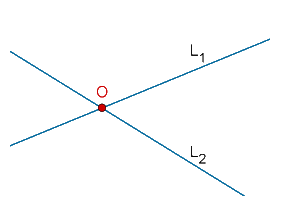A moving point X moves in such a way so that it is equidistant (at the same distance) from L1 and L2. What will be its path, or locus? In other words, how will X move given the constraint that it is at the same distance from L1 and L2?

Recall the case of the perpendicular bisector. In that case, we wanted a point to be always equidistant from two fixed points. Here, we want a point to be always equidistant from two fixed lines. There is a difference between the two.

The answer to our current problem is that X must lie on one of the two angle bisectors of L1 and L2. It shouldn’t be difficult to understand that there will be two angle bisectors, as the figure below shows: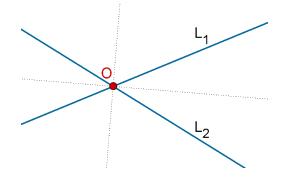Now, suppose that X lies on any one of the two angle bisectors. We then need to show that X is equidistant from L1 and L2. In other words, the perpendiculars from X onto L1 and L2 will be of the same length: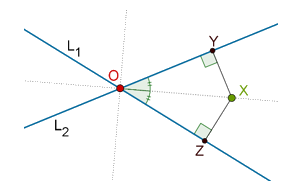We need to show that XY = XZ. Consider the two triangles OXY and OXZ:

1.  OX = OX (common side)

2.  ∠XOY = ∠XOZ (OX is the angle bisector of ang YOZ)

3.  ∠XYO = ∠XZO = 90°

By the ASA criterion, ∆OXY ≡ ∆OXZ, which means that XY = XZ. Thus, X is equidistant from L1 and L2. And this result holds regardless of which of the two angle bisectors X lies on.

To summarize: a point equidistant from two lines must lie on one of the two angle bisectors of the angles formed between the two lines.

Example 1: O is any point inside $$\Delta ABC$$. Prove that $$\angle BOC > \angle BAC$$.

Solution: Consider the following figure: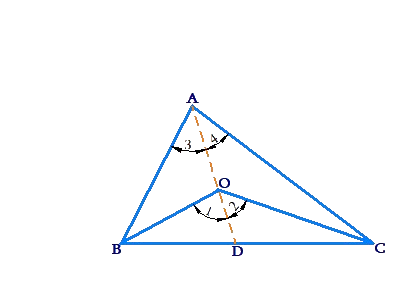Through A, we have drawn AO, which intersects BC at D. We have also marked various angles in the figure.

Note that $$\angle 1$$ is an exterior angle for $$\Delta AOB.$$ Clearly,

$$\angle 1 > \angle 3$$

Similarly,

$$\angle 2 > \angle 4$$

Adding these two inequalities,we have:

$$\begin{array}{l}\angle 1 + \angle 2 > \angle 3 + \angle 4\\ \Rightarrow \;\;\;\angle BOC > \angle BAC\end{array}$$

Example 2: The bisector of $$\angle A$$ of $$\Delta ABC$$ meets BC at D. If $$AB > AC,$$ prove that $$\angle ADC$$ is acute.

Solution: Consider the following figure, which shows $$\Delta ABC$$ with $$AB > AC$$: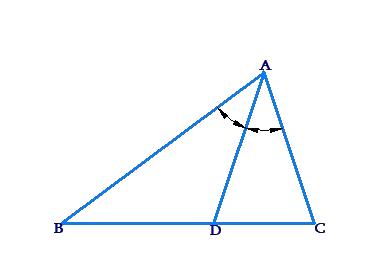Since AB > AC, we have $$\angle C > \angle B$$ (angle opposite the larger side will be larger). Now, we will make use of the exterior angle theorem. We note that

$\angle ADB = \frac{1}{2}\angle A + \angle C$ $\angle ADC = \frac{1}{2}\angle A + \angle B$

Clearly, $$\angle ADB > \angle ADC$$. But since these two angles form a supplementary pair, this further means that $$\angle ADB$$ must be obtuse and $$\angle ADC$$ must be obtuse. Thus, our proof is complete.

Example 3: This problem consists of four parts, which should be covered in sequence

(a)  Let D be any point on side BC of $$\Delta ABC$$. Prove that at least one of AB or AC must be greater than AD.

(b)  Let BC be the greatest side of $$\Delta ABC$$. Suppose that D and E are points on BC and CA    re  spectively. Prove that $$BC \ge DE$$.

(c)  Let BC be the greatest side of $$\Delta ABC$$, and let E and F be points on AB and AC respectively. Prove that $$BC \ge EF$$.

(d)  Prove that no straight line segment can be drawn within a triangle which is greater than its greatest side.

Solution:

(a)  Consider the following figure: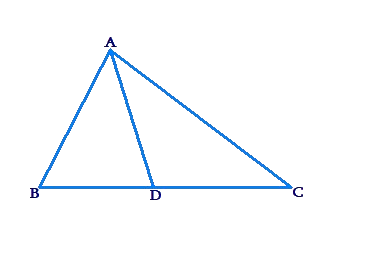Let us suppose that AC > AB. This means that$$\angle B > \angle C$$. Now, using the exterior angle theorem in $$\angle ABD,$$ we see that

$\begin{array}{l}\angle ADC = \angle B + \angle BAD\\ \Rightarrow \;\;\angle ADC > \angle B\end{array}$

But since $$\angle B > \angle C,$$ we have $$\angle ADC > \angle C,$$ which means (in $$\angle ADC$$) that AC > AD.

Similarly, if AB > AC, we can show that AB must be larger than AD. In both cases,at least one of AB or AC must be greater than AD (note that both of them could also possibly be greater than AD).

The proof for the case of AB = AC is left to the reader as an exercise.

(b)  The following figure shows a $$\angle ABC$$ with BC as its largest side. We have also marked D and E as arbitrary locations on BC and CA respectively: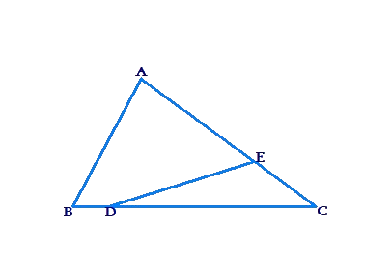We need to prove that $$BC \ge DE,$$ i.e., BC cannot be less than DE. Join A to D: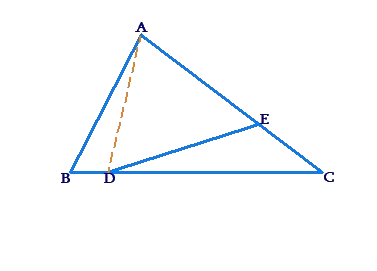Consider $$\Delta ADC$$. Using the result of part-(a), at least one of AD or DC must be greater than DE. Now, we will show that BC is greater than both AD and DC:

• BC is obviously larger than DC.

• Consider $$\Delta ABC$$. AD must be smaller than at least one of AB or AC (part-(a)), and both AB and AC are smaller than BC (since BC is largest side). Thus, AD < BC.

Since BC is greater than both AD and DC, and at least one of (AD, DC) is greater than DE, we conclude that BC > DE.

A special case occurs when D coincides with B and E coincides with C. In this case, BC = DE. Combining the two results, we have:

$BC \ge DE$

(c)  Once again, the following figure shows $$\Delta ABC$$ with BC as the largest side. E and F are points on AB and AC respectively: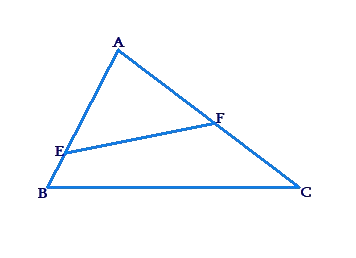We need to show that $$BC \ge EF$$. Some of you may say that this visually obvious. EF can never be larger than BC. However, we need to demonstrate this through a logical, step-by-step proof.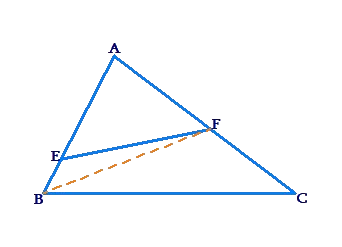Consider $$\Delta AFB$$. EF must be smaller than at least one of AF or BF. We further note that:

• AF < AC < BC, because BC is given to be the largest side.

• In $$\angle ABC$$, BF must be smaller than at least one of (AB, AC). Since BC is the largest side, this means than BF must be less than BC.

Thus, both AF and BF are smaller than BC, and since EF is smaller than at least one of (AF, BF), we conclude that EF < BC.

Note the special case when E coincides with and F with C, in which case EF = BC. Thus, we can say that $$EF \le BC$$.

(d)  Now comes the final and most interesting part of the problem, which builds on the previous part. Consider an arbitrary $$\Delta ABC$$ with BC as its largest side, in which an arbitrary segment XY has been drawn: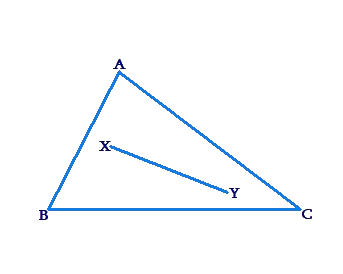We have to show that no matter what, XY cannot be larger than BC. Join A to X and Y, such that AX and AY meet BC at D and E respectively: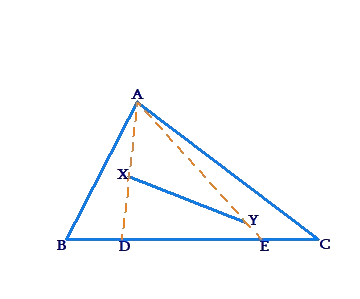Using the result of part-(c), we have $$XY \le DE$$. But obviously, $$DE \le BC.$$ Equality occurs if X coincides with B and Y coincides with C.

Example 4: In any $$\Delta ABC$$, prove that the bisector of the interior angle A and the exterior angles at B and C will be concurrent.

Solution: Consider the following figure, in which the bisectors of the exterior angles at B and C meet at X. We will prove that AX is the bisector of angle A.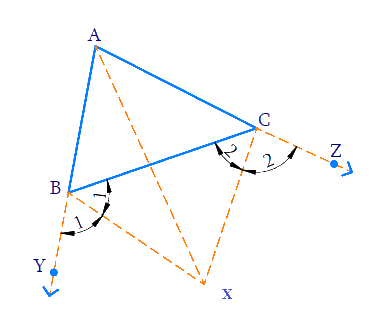• Since X lies on the angle bisector of$$\angle YBC$$, it is equidistant from BY and BC (the perpendicular distances of X from the two segments or segments-extended will be the same).
• Similarly, X must be equidistant from CZ and BC.

From these two observations, we conclude that X must be equidistant from BY and CZ, i.e., from AY and AZ. This means that X must lie on the angle bisector of $$\angle YAZ$$, that is, AX is the angle bisector of $$\angle A$$. This completes our proof.